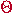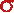Math & Science Home | Proficiency Tests | Mathematical Thinking in Physics | Aeronauts 2000

 CONTENTS Introduction Fermi's Piano Tuner Problem How Old is Old? If the Terrestrial Poles were to Melt... Sunlight Exerts Pressure Falling Eastward What if an Asteroid Hit the Earth Using a Jeep to Estimate the Energy in Gasoline How do Police Radars really work? How "Fast" is the Speed of Light? How Long is a Light Year? How Big is a Trillion? "Seeing" the Earth, Moon, and Sun to Scale Of Stars and Drops of Water If I Were to Build a Model of the Cosmos... A Number Trick Designing a High Altitude Balloon Pressure in the Vicinity of a Lunar Astronaut Space Suit due to Outgassing of Coolant Water Calendar Calculations Telling Time by the Stars - Sidereal Time Fields, an Heuristic Approach The Irrationality ofThe Irrationality ofThe Number (i)i Estimating the Temperature of a Flat Plate in Low Earth Orbit Proving that (p)1/n is Irrational when p is a Prime and n>1 The Transcendentality ofIdeal Gases under Constant Volume, Constant Pressure, Constant Temperature and Adiabatic Conditions Maxwell's Equations: The Vector and Scalar Potentials A Possible Scalar Term Describing Energy Density in the Gravitational Field A Proposed Relativistic, Thermodynamic Four-Vector Motivational Argument for the Expression-eix=cosx+isinx Another Motivational Argument for the Expression-eix=cosx+isinx Calculating the Energy from Sunlight over a 12 hour period Calculating the Energy from Sunlight over actual full day Perfect Numbers-A Case Study Gravitation Inside a Uniform Hollow Sphere Further note on Gravitation Inside a Uniform Hollow Sphere Pythagorean Triples Black Holes and Point Set Topology Additional Notes on Black Holes and Point Set Topology Field Equations and Equations of Motion (General Relativity) The observer in modern physics A Note on the Centrifugal and Coriolis Accelerations as Pseudo Accelerations - PDF File On Expansion of the Universe - PDF File

##### Factoring in the Actual Length of Day for Solar Energy Calculations

It can be shown that if TD is the length of day - i.e., the time in hours from sunrise to sunset (not taking into account the refraction of the earth's atmosphere) - then TD satisfies

cos(TD /24) = -(tan)(tan L)

whereis the sun's position north of the equator (the solar declination) and L is the observer's latitude. (This equation can be obtained by a careful application of spherical trigonometry that will not be given here.) Solving for TD gives

TD = (24/) arccos {(-tan)(tan L)} hr.

In our previous article on collecting energy from the sun, the assumption was made that energy could be collected over a 12-hour period. In fact, this assumption is not adequate since solar energy can be collected only over the period of actual daylight. Thus, in the expression

dt = (12/) dA hr

we should replace the factor 12 with TD to give

dt = (TD /) dA hr.

Making this substitution gives for the energy E collected over an actual daylight period TD

dE =D (sin A) (TD /) (3,600 sec/hr) dA
=D (3,600 TD /) [(sin A) dA]
and
E =D (3,600 TD /).

Now, let's complete the midsummer and midwinter calculations again and compare the results with those obtained previously.

1. As before, L = 42°. At midsummer,= 23.5°; at midwinter,= -23.5°.
2. In midsummer,D = (137mW/cm2) cos (42° - 23.5°) = 130 mW/cm2.
3. In midsummer,
TD = (24/) arccos {(-tan 23.5°)(tan 42°)} Hr = 15 hr.
4. The energy collected by our 1 cm2 collector is
E = (130 mW/cm2)(3,600sec/hr)(15hr/) = 2.2 x 106 mJ.
5. In midwinter,D = (137mW/cm2) cos (42° + 23.5°) = 56.8 mW/cm2.
6. In mid winter,
TD = (24/) arccos {(-tan (-23.5°))(tan 42°)} Hr = 8.9 Hr.
7. The energy collected by our 1 cm2 collector is
E = (56.8 mW/cm2)(3,600 sec/hr)( 8.9 hr/) = 5.8 x 105 mJ.

The ratio between midsummer and midwinter is

(5.8 x 105 mJ)/(2.2 x 106 mJ) = 0.26

which means that over an actual daylight period my solar collector actually collects 26% of the energy d in midwinter that it would in midsummer. (No wonder our winters are soooooooo cold!!!)

Physicists often make the kinds of approximations that you have seen in these two articles. The comparison of results between the 12-hour day and the actual length of day approximations shows the level of care that always must be exercised.

Please send suggestions/corrections to:
Web Related: David.Mazza@grc.nasa.gov
Technology Related: Joseph.C.Kolecki@grc.nasa.gov
Responsible NASA Official: Theresa.M.Scott (Acting)